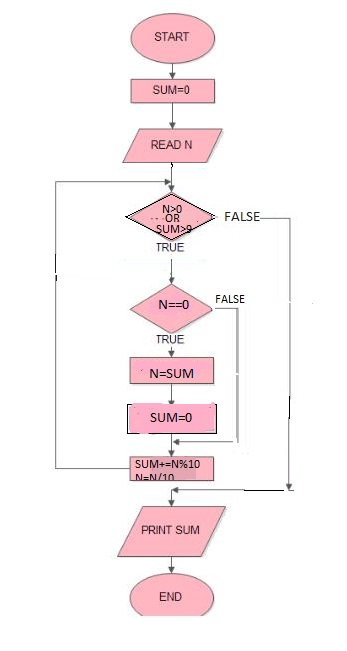Related Articles
Finding sum of digits of a number until sum becomes single digit
• Difficulty Level : Medium
• Last Updated : 26 Mar, 2021

Given a number n, we need to find the sum of its digits such that:

```If n < 10
digSum(n) = n
Else
digSum(n) = Sum(digSum(n))```

Examples :

```Input : 1234
Output : 1
Explanation : The sum of 1+2+3+4 = 10,
digSum(x) == 10
Hence ans will be 1+0 = 1

Input : 5674
Output : 4 ```

A brute force approach is to sum all the digits until sum < 10.
Flowchart:Below is the brute force program to find the sum.

## C++

 `// C++ program to find sum of``// digits of a number until``// sum becomes single digit.``#include`` ` `using` `namespace` `std;` `int` `digSum(``int` `n)``{``    ``int` `sum = 0;``   ` `    ``// Loop to do sum while``    ``// sum is not less than``    ``// or equal to 9``    ``while``(n > 0 || sum > 9)``    ``{``        ``if``(n == 0)``        ``{``            ``n = sum;``            ``sum = 0;``        ``}``        ``sum += n % 10;``        ``n /= 10;``    ``}``    ``return` `sum;``}` `// Driver program to test the above function``int` `main()``{``    ``int` `n = 1234;``    ``cout << digSum(n);``    ``return` `0;``}`

## Java

 `// Java program to find sum of``// digits of a number until``// sum becomes single digit.``import` `java.util.*;` `public` `class` `GfG {``    ` `    ``static` `int` `digSum(``int` `n)``    ``{``        ``int` `sum = ``0``;` `        ``// Loop to do sum while``        ``// sum is not less than``        ``// or equal to 9``        ``while` `(n > ``0` `|| sum > ``9``)``        ``{``            ``if` `(n == ``0``) {``                ``n = sum;``                ``sum = ``0``;``            ``}``            ``sum += n % ``10``;``            ``n /= ``10``;``        ``}``        ``return` `sum;``    ``}``    ` `    ``// Driver code``    ``public` `static` `void` `main(String argc[])``    ``{``        ``int` `n = ``1234``;``        ``System.out.println(digSum(n));``    ``}``}` `// This code is contributed by Gitanjali.`

## Python

 `# Python program to find sum of``# digits of a number until``# sum becomes single digit.``import` `math` `# method to find sum of digits``# of a number until sum becomes``# single digit``def` `digSum( n):``    ``sum` `=` `0``    ` `    ``while``(n > ``0` `or` `sum` `> ``9``):``    ` `        ``if``(n ``=``=` `0``):``            ``n ``=` `sum``            ``sum` `=` `0``        ` `        ``sum` `+``=` `n ``%` `10``        ``n ``/``=` `10``    ` `    ``return` `sum` `# Driver method``n ``=` `1234``print` `(digSum(n))` `# This code is contributed by Gitanjali.`

## C#

 `// C# program to find sum of``// digits of a number until``// sum becomes single digit.``using` `System;` `class` `GFG {``    ` `    ``static` `int` `digSum(``int` `n)``    ``{``        ``int` `sum = 0;` `        ``// Loop to do sum while``        ``// sum is not less than``        ``// or equal to 9``        ``while` `(n > 0 || sum > 9)``        ``{``            ``if` `(n == 0)``            ``{``                ``n = sum;``                ``sum = 0;``            ``}``            ``sum += n % 10;``            ``n /= 10;``        ``}``        ``return` `sum;``    ``}``    ` `    ``// Driver code``    ``public` `static` `void` `Main()``    ``{``        ``int` `n = 1234;``        ``Console.Write(digSum(n));``    ``}``}` `// This code is contributed by nitin mittal`

## PHP

 ` 0 || ``\$sum` `> 9)``    ``{``        ``if``(``\$n` `== 0)``        ``{``            ``\$n` `= ``\$sum``;``            ``\$sum` `= 0;``        ``}``        ``\$sum` `+= ``\$n` `% 10;``        ``\$n` `= (int)``\$n` `/ 10;``    ``}``    ``return` `\$sum``;``}` `// Driver Code``\$n` `= 1234;``echo` `digSum(``\$n``);` `// This code is contributed``// by aj_36``?>`

## Javascript

 ``

Output :

`1`

There exists a simple and elegant O(1) solution for this too. The ans is given by simply :-

```If n == 0
return 0;

If n % 9 == 0
digSum(n) = 9
Else
digSum(n) = n % 9 ```

How does the above logic works?
If a number n is divisible by 9, then the sum of its digit until sum becomes single digit is always 9. For example,
Let, n = 2880
Sum of digits = 2 + 8 + 8 = 18: 18 = 1 + 8 = 9
A number can be of the form 9x or 9x + k. For the first case, answer is always 9. For the second case, and is always k.

Below is the implementation of the above idea :

## C++

 `#include``using` `namespace` `std;` `int` `digSum(``int` `n)``{``    ``if` `(n == 0)``       ``return` `0;``    ``return` `(n % 9 == 0) ? 9 : (n % 9);``}` `// Driver program to test the above function``int` `main()``{``    ``int` `n = 9999;``    ``cout<

## Java

 `import` `java.io.*;` `class` `GFG {` `    ``static` `int` `digSum(``int` `n)``    ``{``        ``if` `(n == ``0``)``        ``return` `0``;``        ``return` `(n % ``9` `== ``0``) ? ``9` `: (n % ``9``);``    ``}``    ` `    ``// Driver program to test the above function``    ``public` `static` `void` `main (String[] args)``    ``{``        ``int` `n = ``9999``;``        ``System.out.println(digSum(n));``    ``}``}` `// This code is contributed by anuj_67.`

## Python3

 `def` `digSum(n):` `    ``if` `(n ``=``=` `0``):``        ``return` `0``    ``if` `(n ``%` `9` `=``=` `0``):``        ``return` `9``    ``else``:``        ``(n ``%` `9``)` `# Driver program to test the above function``n ``=` `9999``print``(digSum(n))` `# This code is contributed by``# Smitha Dinesh Semwal`

## C#

 `using` `System;` `class` `GFG``{``    ``static` `int` `digSum(``int` `n)``    ``{``        ``if` `(n == 0)``        ``return` `0;``        ``return` `(n % 9 == 0) ? 9 : (n % 9);``    ``}``    ` `    ``// Driver Code``    ``public` `static` `void` `Main ()``    ``{``        ``int` `n = 9999;``        ``Console.Write(digSum(n));``    ` `    ``}``}` `// This code is contributed by aj_36`

## PHP

 ``

## Javascript

 ``

Output:

`9`

This article is contributed by Ayush Khanduri. If you like GeeksforGeeks and would like to contribute, you can also write an article using contribute.geeksforgeeks.org or mail your article to contribute@geeksforgeeks.org. See your article appearing on the GeeksforGeeks main page and help other Geeks.
Please write comments if you find anything incorrect, or you want to share more information about the topic discussed above.

Attention reader! Don’t stop learning now. Get hold of all the important DSA concepts with the DSA Self Paced Course at a student-friendly price and become industry ready.

My Personal Notes arrow_drop_up